Courses

# Please go through following and answer question no.7 to no.8Each of the following questions consists of five Problem figures marked 1,2,3,4 and 5 followed by five Answer figures marked A,B,C,D and E . Select a figure from the Answer figures which will continue the same series as given in the Problem figures.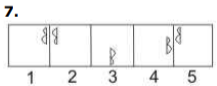a)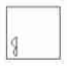b)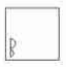c)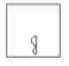d)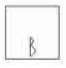e)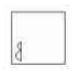Correct answer is option 'A'. Can you explain this answer? Related Test: BITSAT Logical Reasoning Test - 1

## JEE Question

By Ganesh Shinde · 4 weeks ago ·JEE
Rajesh Mhatre answered 4 weeks ago
A is correct

Shubham Rajput answered May 11, 2019
Yes a is correct Yes the correct answer is a as figures are alternate and are moving in anticlockwise direction

Anand Rai answered 4 weeks ago
I think option E is also right

Upma Sharma answered Apr 11, 2019
The symbol gets vertically inverted and laterally inverted alternately. It also moves in ACW direction through distances equal to two half-sides (of square boundary) and three halfsides alternately